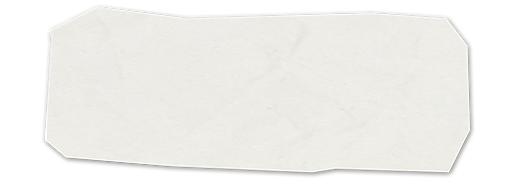## 8. Chemical Equations

8.1 Converting amount↔moles↔mass AND calculating percent composition.

8.2 Determining empirical formulas

8.3 Balancing Equations

8.4 The Law of Conservation of Mass.  Chemistry review practice which includes diatomic elements, ionic compounds, balancing equations, dimensional analysis, and gram-mole conversions

8.5 Reaction types